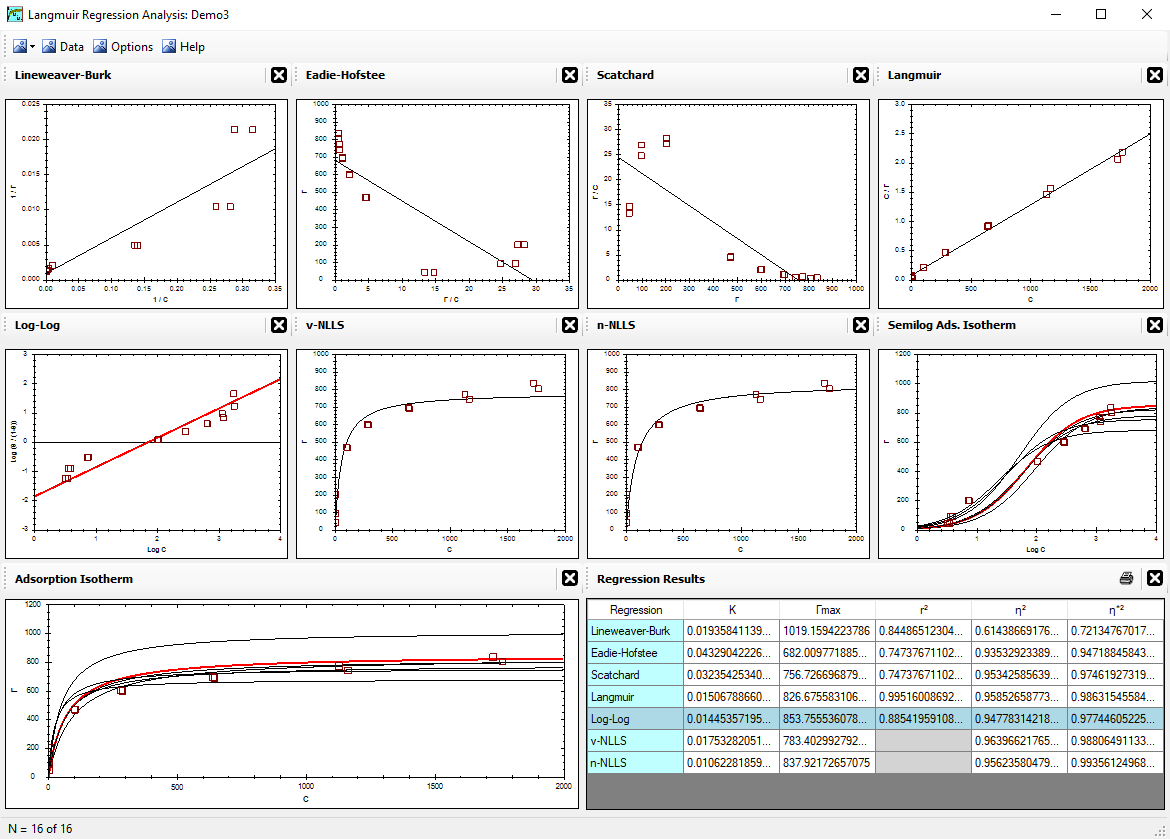Alfisol, Item 003: LMMpro, version 2.0 The Langmuir Optimization Program plus The Michaelis-Menten Optimization ProgramWhat does LMMpro do? List of Published Articles Using LMMpro History of Linear Regression Methods Linear Regressions Deriving Linear Regression Methods

## History of Linear Regression Methods for Langmuir & Michaelis-Menten Equations.

The Langmuir and Michaelis-Menten Equations are very similar to each other in form. They are both parabolic equations. Accordingly, the methods developed to solve these equations are also similar. The objective is to find the best K and Γmax values of the Langmuir Equation, or the best KM and Vmax values of the Michaelis-Menten Equation, so that the parabolic equation fits the data with minimal error.

There are several methods available. Without computers, it is best to convert the parabolic equation into a linear form, which can then be solved by linear regression techniques. Langmuir proposed a linearization method in 1918 (known as the Langmuir linear regression), but it was not known to those using the Michaelis-Menten Equation. Accordingly, Hanes and later Woolf proposed a similar regression technique in 1932 (known as the Hanes-Woolf linear regression). Note that the Langmuir linear regression and the Hanes-Woolf linear regression are identical in form.

A common method used to solve the Michaelis-Menten Equation is the Lineweaver-Burk linear regression. Since several early environmental scientists did not know about Langmuir's linearization methods, this Lineweaver-Burk linear regression was also used extensively to solve the Langmuir Equation. This method is very sensitive to data error, and its use is now strongly discouraged.

A few years later, Eadie-Hofstee (1942, 1952) proposed another method for solving the Michaelis-Menten Equation. The Eadie-Hofstee linear regression was a significant improvement from the earlier Lineweaver-Burk linear regression, but it is still somewhat sensitive to data error. Once again, several early environmental scientists did not know about Langmuir's linearization method, and this Eadie-Hofstee linear regression was also used extensively to solve the Langmuir Equation.

Another effort to come up with a better Michaelis-Menten optimization method was presented by Scatchard in 1949. It still suffers from some sensitivity to data error. Once again, several early environmental scientists did not know about Langmuir's linearization method, and this Scatchard linear regression was also used extensively to solve the Langmuir Equation.

Finally, the best Michaelis-Menten optimization method using linear regression techniques was presented by Hanes 1932, and was referred to as the Hanes-Woolf regression by Haldane (1957). As noted earlier, this technique is essentially identical to the linear regression technique presented by Langmuir in 1918. It is the least sensitive to data error. Once again, several early environmental scientists did not know about Langmuir's linearization methods, and this Hanes-Woolf linear regression was also used extensively to solve the Langmuir Equation. That is, the technique was incorrectly named the Hanes-Woolf linear regression (rather than the Langmuir linear regression) when used to solve the Langmuir Equation.

There is one more linear regression that is worth noting here. The data can be presented in a log-log form, resulting in a linear pattern with a slope = 1.0. It is optimized via an iteration process, and, hence, this method is not used. That is, this method is not easy to use without computers.

<Reference = CTT-4>